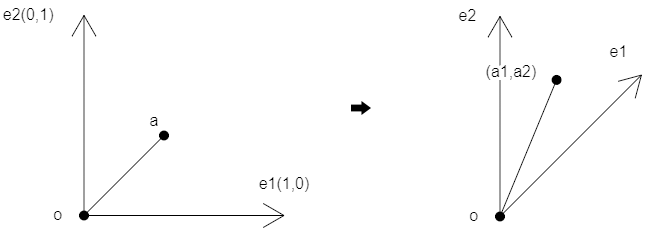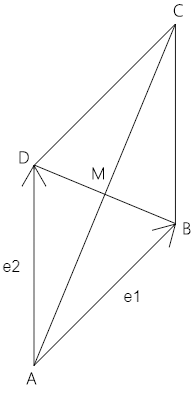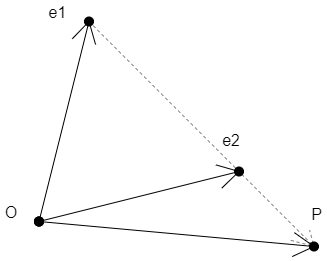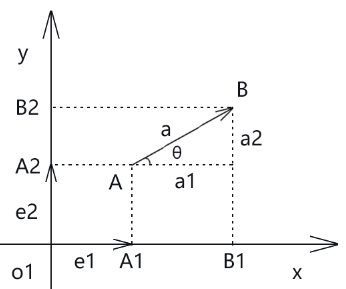## 第一章 向量在二维坐标系里的姿态

### 1-平面向量基本定理

• 平面内存在一个向量a
• 平面内存在e1、e2 两个不平行的向量

• 该平面内存在唯一一对实数a1、a2，使：a=a1e1+a2e2

### 2-平面向量的特性

e1,e2 ：二维坐标系里的两个基底，记做{e1,e2}。

a1e1+a2e2 ：向量a关于基底{e1,e2} 的分解式。

• 点a在二维直角坐标系中。

• (a1,a2) 可以视之为一个点位，它处于以{e1,e2} 为基底的坐标系中。### 3-示例• 平行四边形ABCD
• 向量AB=e1

``````AC=AB+AD=e1+e2
AM=AC/2
AM=(e1+e2)/2
AM=e1/2+e2/2
``````

### 4-向量的参数方程式

``````OP=(1-t)*OA+t*OB
``````

``````OP=OA-t*OA+t*OB
OP=OA+(OB-OA)*t
````````````e1P=t*(e1e2)=t*(e2-e1)
OP=e1+e1P
=e1+t*(e2-e1)
=e1+t*e2-t*e1
=(1-t)*e1+t*e2
``````

### 2- 用向量的观点认识直角坐标系``````a=a1*e1+a2*e2
``````

a1、a2就是向量a 在基底{e1,e2}下的坐标，即：

``````a=(a1,a2)
``````
• a1：向量a 在x 轴上的坐标分量
• a2：向量a 在y 轴上的坐标分量

• 坐标分量a1 为向量A1B1 在x 轴上的坐标投影
• 坐标分量a2 为向量A2B2 在y 轴上的坐标投影

``````O =(0,0)
e1=(1,0)
e2=(0,1)
``````

``````a1=|a|*cosθ
a2=|a|*sinθ
``````

### 3-(x,y)的双重意义

• e1=一个像素的宽
• e2=负的一个像素的高

``````OA=x*e1+y*e2
OA=(x,y)
``````

• e1=(1,0)
• e2=(0,1)
• a1=3
• a2=2

``````a=3*(1,0)+2*(0,1)
=(3,0)+(0,2)
=(3,2)
``````

### 1-向量运算的基本概念

• 两个向量和与差的坐标等于两个向量相应坐标分量的和与差。
• 向量的数乘等于此数乘以向量的坐标分量。
• 一个向量的坐标等于向量终点的坐标减去向量始点的坐标。

### 2-两个向量平行的条件

• 基底为{e1,e2}
• a=(a1,a2)
• b=(b1,b2)

a=λb 可分解为：

``````(a1,a2)=λ(b1,b2)=(λb1,λb2)
``````

``````a1=λb1
a2=λb2``````

## 发表评论

•• 匿名